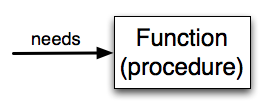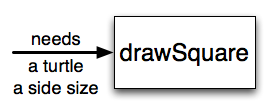# 6.1. Functions¶

Video: (function_intro)

In Python, a function is a named sequence of statements that belong together. Their primary purpose is to help us organize programs into chunks that match how we think about the solution to the problem.

The syntax for a function definition is:

def name( parameters ):
statements


You can make up any names you want for the functions you create, except that you can’t use a name that is a Python keyword, and the names must follow the rules for legal identifiers that were given previously. The parameters specify what information, if any, you have to provide in order to use the new function. Another way to say this is that the parameters specify what the function needs to do its work.

There can be any number of statements inside the function, but they have to be indented from the def. In the examples in this book, we will use the standard indentation of four spaces. Function definitions are the second of several compound statements we will see, all of which have the same pattern:

1. A header line which begins with a keyword and ends with a colon.

2. A body consisting of one or more Python statements, each indented the same amount – 4 spaces is the Python standard – from the header line.

We’ve already seen the for loop which follows this pattern.

In a function definition, the keyword in the header is def, which is followed by the name of the function and some parameters enclosed in parentheses. The parameter list may be empty, or it may contain any number of parameters separated from one another by commas. In either case, the parentheses are required.

We need to say a bit more about the parameters. In the definition, the parameter list is more specifically known as the formal parameters. This list of names describes those things that the function will need to receive from the user of the function. When you use a function, you provide values to the formal parameters.

The figure below shows this relationship. A function needs certain information to do its work. These values, often called arguments or actual parameters, are passed to the function by the user.This type of diagram is often called a black-box diagram because it only states the requirements from the perspective of the user. The user must know the name of the function and what arguments need to be passed. The details of how the function works are hidden inside the “black-box”.

Suppose we’re working with turtles and a common operation we need is to draw squares. It would make sense if we did not have to duplicate all the steps each time we want to make a square. “Draw a square” can be thought of as an abstraction of a number of smaller steps. We will need to provide two pieces of information for the function to do its work: a turtle to do the drawing and a size for the side of the square. We could represent this using the following black-box diagram.Here is a program containing a function to capture this idea. Give it a try.

This function is named drawSquare. It has two parameters — one to tell the function which turtle to move around and the other to tell it the size of the square we want drawn. In the function definition they are called t and sz respectively. Make sure you know where the body of the function ends — it depends on the indentation and the blank lines don’t count for this purpose!

docstrings

If the first thing after the function header is a string (some tools insist that it must be a triple-quoted string), it is called a docstring and gets special treatment in Python and in some of the programming tools.

Another way to retrieve this information is to use the interactive interpreter, and enter the expression <function_name>.__doc__, which will retrieve the docstring for the function. So the string you write as documentation at the start of a function is retrievable by python tools at runtime. This is different from comments in your code, which are completely eliminated when the program is parsed.

By convention, Python programmers use docstrings for the key documentation of their functions.

Defining a new function does not make the function run. To do that we need a function call. This is also known as a function invocation. We’ve already seen how to call some built-in functions like print, range and int. Function calls contain the name of the function to be executed followed by a list of values in parentheses, called arguments, which are assigned to the parameters in the function definition. So in the second to the last line of the program, we call the function, and pass alex as the turtle to be manipulated, and 50 as the size of the square we want.

Once we’ve defined a function, we can call it as often as we like and its statements will be executed each time we call it. In this case, we could use it to get one of our turtles to draw a square and then we can move the turtle and have it draw a different square in a different location. Note that we lift the tail so that when alex moves there is no trace. We put the tail back down before drawing the next square. Make sure you can identify both invocations of the drawSquare function.

In the next example, we’ve changed the drawSquare function a little and we get tess to draw 15 squares with some variations. Once the function has been defined, we can call it as many times as we like with whatever actual parameters we like.

Warning

Even if a function call needs no arguments, the parentheses ( ) after the function name are required. This can lead to a difficult bug: A function name without the parenthesis is a legal expression referring to the function; for example, print and alex.penup, but they do not call the associated functions.

Note

This workspace is provided for your convenience. You can use this activecode window to try out anything you like.

func-1-1: What is a function in Python?

• A named sequence of statements.
• Yes, a function is a named sequence of statements.
• Any sequence of statements.
• While functions contain sequences of statements, not all sequences of statements are considered functions.
• A mathematical expression that calculates a value.
• While some functions do calculate values, the python idea of a function is slightly different from the mathematical idea of a function in that not all functions calculate values. Consider, for example, the turtle functions in this section. They made the turtle draw a specific shape, rather than calculating a value.
• A statement of the form x = 5 + 4.
• This statement is called an assignment statement. It assigns the value on the right (9), to the name on the left (x).

func-1-2: What is one main purpose of a function?

• To improve the speed of execution
• Functions have little effect on how fast the program runs.
• To help the programmer organize programs into chunks that match how they think about the solution to the problem.
• While functions are not required, they help the programmer better think about the solution by organizing pieces of the solution into logical chunks that can be reused.
• All Python programs must be written using functions
• In the first several chapters, you have seen many examples of Python programs written without the use of functions. While writing and using functions is desirable and essential for good programming style as your programs get longer, it is not required.
• To calculate values.
• Not all functions calculate values.

func-1-3: Which of the following is a valid function header (first line of a function definition)?

• def drawCircle(t):
• A function may take zero or more parameters. It does not have to have two. In this case the size of the circle might be specified in the body of the function.
• def drawCircle:
• A function needs to specify its parameters in its header.
• drawCircle(t, sz):
• A function definition needs to include the keyword def.
• def drawCircle(t, sz)
• A function definition header must end in a colon (:).

func-1-4: What is the name of the following function?

def drawSquare(t, sz):
"""Make turtle t draw a square of with side sz."""
for i in range(4):
t.forward(sz)
t.left(90)

• def drawSquare(t, sz)
• This line is the complete function header (except for the semi-colon) which includes the name as well as several other components.
• drawSquare
• Yes, the name of the function is given after the keyword def and before the list of parameters.
• drawSquare(t, sz)
• This includes the function name and its parameters
• Make turtle t draw a square with side sz.
• This is a comment stating what the function does.

func-1-5: What are the parameters of the following function?

def drawSquare(t, sz):
"""Make turtle t draw a square of with side sz."""
for i in range(4):
t.forward(sz)
t.left(90)

• i
• i is a variable used inside of the function, but not a parameter, which is passed in to the function.
• t
• t is only one of the parameters to this function.
• t, sz
• Yes, the function specifies two parameters: t and sz.
• t, sz, i
• the parameters include only those variables whose values that the function expects to receive as input. They are specified in the header of the function.

func-1-6: Considering the function below, which of the following statements correctly invokes, or calls, this function (i.e., causes it to run)? Assume we already have a turtle named alex.

def drawSquare(t, sz):
"""Make turtle t draw a square of with side sz."""
for i in range(4):
t.forward(sz)
t.left(90)

• def drawSquare(t, sz)
• No, t and sz are the names of the formal parameters to this function. When the function is called, it requires actual values to be passed in.
• drawSquare
• A function call always requires parentheses after the name of the function.
• drawSquare(10)
• This function takes two parameters (arguments)
• drawSquare(alex, 10):
• A colon is only required in a function definition. It will cause an error with a function call.
• drawSquare(alex, 10)
• Since alex was already previously defined and 10 is a value, we have passed in two correct values for this function.

func-1-7: True or false: A function can be called several times by placing a function call in the body of a loop.

• True
• Yes, you can call a function multiple times by putting the call in a loop.
• False
• One of the purposes of a function is to allow you to call it more than once. Placing it in a loop allows it to executed multiple times as the body of the loop runs multiple times.